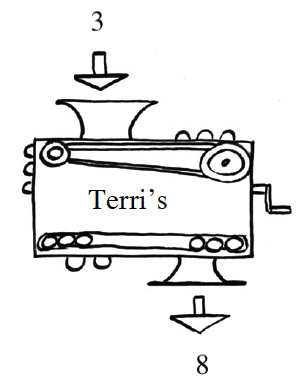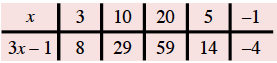Home > INT3 > Chapter 1 > Lesson 1.1.2 > Problem1-18

1-18.Terri’s project for the Math Fair is a magnificent black box that she called a function machine. If you put $3$ into her machine, the output is $8$. If you put in $10$, the output is $29$; and if you put in $20$, the output is $59$. Homework Help ✎

1. What would her machine do to an input of $5$? What about $-1$? What about $x$? Making an input → output table may help.

Look for a pattern.

Try making a table and graphing the points.

If the input is $x$, what is the output?2. Write an equation for Terri's machine.

$f(x)=3x−1$